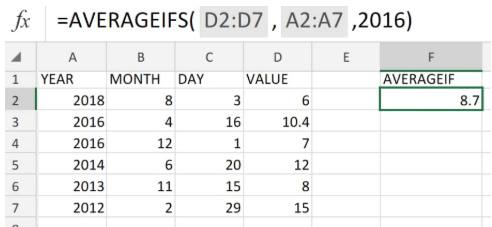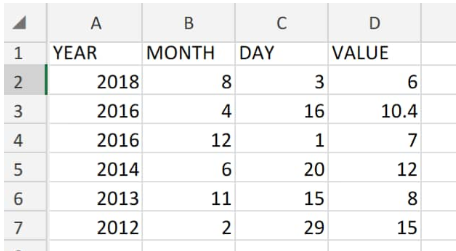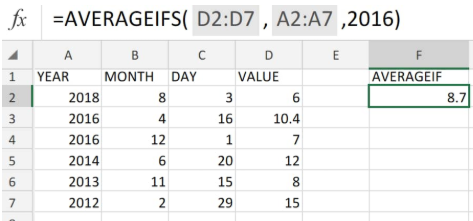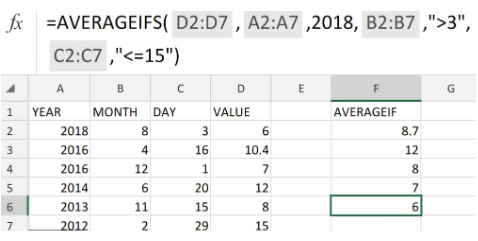Get instant live expert help with Excel or Google Sheets“My Excelchat expert helped me in less than 20 minutes, saving me what would have been 5 hours of work!”

#### Post your problem and you’ll get expert help in seconds.

Your message must be at least 40 characters
Our professional experts are available now. Your privacy is guaranteed.

# AVERAGEIF function in Excel – Excelchat

The AVERAGEIF function in Excel determines the average of a range of numbers that meets a given criteria.

The AVERAGEIF is an in-built Excel function which is categorized under its Statistical Functions.Figure 1. of AVERGEIF Function in Excel

We can utilize the AVERAGEIF function in excel as a worksheet function (WS) since the average ifs may be included as a formula component within a worksheet cell.

## Formula Syntax

`=AVERAGEIF (range, criteria, [average_range])`

• range = Amount of cells, containing number values or names, references, or arrays.
• criteria = An expression, number, text, or cell reference.
• average_range (optional) = The cells we wish to average. When this is omitted, the range of cells is used.

## How to use AVERAGEIF in Excel

Avgif Excel is a straightforward and user-friendly function.

1. To find out the average of certain number ranges, we can start by arranging them in labelled columns of our worksheet.Figure 2. of Number Ranges for AVERAGEIF in Excel

Our goal here is to use AVERAGEIF formulas to test if the average value of the range of numbers in column D meets our criteria in column A of our worksheet example;

The AVERAGEIF formula we will enter into cell F2 of our worksheet is as follows;

`=AVERAGEIFS(D2:D7,A2:A7,2016)`Figure 3. of AVERAGEIF Function in Excel

Based on the Excel spreadsheet above, we can generate a variety of averageif formulas to test other average values against our criteria.

The AVERAGEIFS examples below would return;Figure 4. of AVERAGEIF Function in ExcelFigure 5. of AVERAGEIF Function in Excel